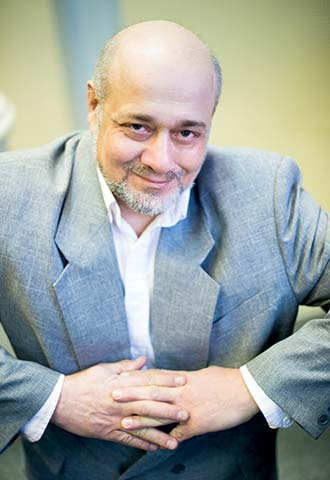# Ilya Pevzner

### ScheduleRecipient of the Francysk Skaryna Medal (2021)

Ilya Pevzner graduated from the Belarusian State Academy of Music in 1994 (class of the People's Artist of Belarus, Professor A.Savchenko).В

He appeared as Don Magnifico in a concert version of La Cenerentola by Gioachino Rossini and in Anna KozlovaвЂ™s musical At the Radziwill Castle, or A Story of Eternal Love at the Belarusian State Philharmonic.В

### REPERTOIRE

The NoblemanВ В В В В В В В В В В В В В В В В В В В В В В В В В  King StakhвЂ™s Wild Hunt by Vladimir Soltan

GeppettoВ  В  В  В  В  В  В  В  В  В  В  В  В  В В В В В В В  В В Pinocchio by Gloria Bruni
FrankВ В В В В В В В В В В В В В В В В В В В В В В В В В В В В В В В В В В В В В В В  Die Fledermaus

The Old GypsyВ В В В В В В В В В В В В В В В В В В В В В В В В  Il trovatore

Dottore, CommissionerВ В В В  В В В В В В В В La traviata

Colline, SergeantВ В В В В В В В В В В В В В В В В В В В В В  La bohГЁme

The MandarinВ В В В В В В В В В В В В В В В В В В В В В В В В В В  Turandot

SamuelВ В В В В В В В В В В В В В В В В В В В В В В В В В В В В В В В В В В В В В  Un ballo in maschera

The JailerВ В В В В В В В В В В В В В В В В В В В В В В В В В В В В В В В В В  Tosca

The CommissionerВ В В В В В В В В В В В В В В В В В В В  Madama Butterfly

Tobias Mill В В В В В В В В В В В В В В В В В В В В В В В В В В В В В В В В La cambiale di matrimonio

Don BasilioВ В В В В В В В В В В В В В В В В В В В В В В В В В В В В В В В  Il barbiere di Siviglia

TonioВ В В В В В В В В В В В В В В В В В В В В В В В В В В В В В В В В В В В В В В В В В  Pagliacci

EscamilloВ В В В В В В В В В В В В В В В В В В В В В В В В В В В В В В В В В В  Carmen

Ibn-HakiaВ В В В В В В В В В В В В В В В В В В В В В В В В В В В В В В В В В В  Iolanta
The TsarвЂ™s StokerВ В В В В В В В В В В В В В В В В В В В В В В  The Tsar's Bride
PoprishchinВ В В В В В В В В В В В В В В В В В В В В В В В В В В В В В В В  В The Diary of a Madman by Vyacheslav Kuznetsov

The Doctor В В В В В В В В В В В В В В В В В В В В В В В В В В В В В В В В В В Magic Music by Mark Minkov

GuillaumeВ В В В В В В В В В В В В В В В В  В В В В В В В В В В В В В В В В В В The Town of Craftsmen by Gennady Gladkov

The OwlВ В В В В В В В В В В В В В В В В В В В В В В В В В В В В В В В В В В В В В  Winnie-the-Pooh by Olga Petrova

The HippopotamusВ В В В В В В В В В В В В В В В В В В В В В  Doctor Ouchithurts by Marina Morozova

Second Priest, Armoured ManВ В В В  Die ZauberflГ¶te

JewВ В В В В В В В В В В В В В В В В В В В В В В В В В В В В В В В В В В В В В В В В В В В В В  Salome

The NotaryВ В В В В В В В В В В В В В В В В В В В В В В В В В В В В В В В В В  Don Pasquale

### CONCERT TOUR

He appeared as Don Magnifico in a concert version of La Cenerentola by Gioachino Rossini at the Belarusian State Philharmonic.

With the company, he has toured Brazil, Germany, Israel, Spain, Italy, Cyprus, Portugal, Thailand, Switzerland, the Netherlands, and the UK.

### Partners